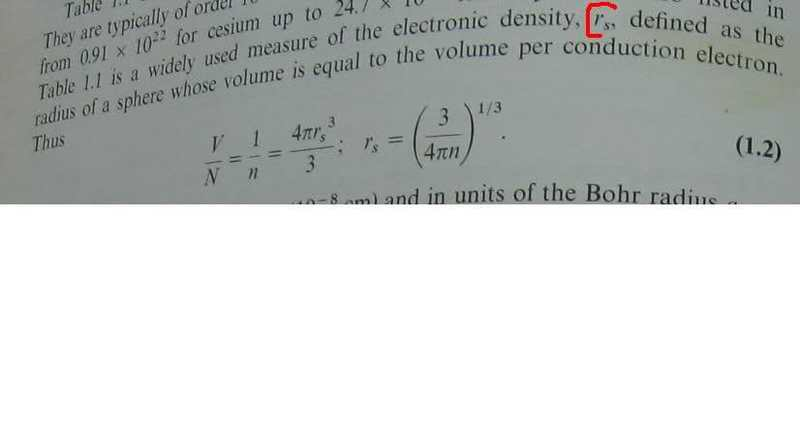# What is r_s ?r_s is the radius of a sphere volume equals ...
what is volume per conduction electron?

what is this volume like?

what is this volume like?

Volume is like another other volume, it's a 3D region of space. In this case the region is taken to be sphere of radius r_n. And within that volume you have N number of conduction electrons.

Divide that and you get a density of conduction electrons per volume or vice-versa.

"Divide that and you get a density of conduction electrons per volume or vice-versa."

divide what?and by what?
density is a mass divided by volume
there is no such thing here

sophiecentaur